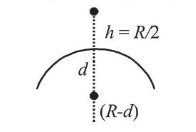# The value of acceleration due to gravityQuestion:

The value of acceleration due to gravity is $g_{1}$ at a height

$h=\frac{R}{2}(R=$ radius of the earth $)$ from the surface of the earth. It is again equal to $g_{1}$ and a depth $d$ below the surface

of the earth. The ratio $\left(\frac{d}{R}\right)$ equals :

1. (1) $\frac{4}{9}$

2. (2) $\frac{5}{9}$

3. (3) $\frac{1}{3}$

4. (4) $\frac{7}{9}$

Correct Option: , 2

Solution:

(2) According to question, $g_{h}=g_{d}=g_{1}$$g_{h}=\frac{G M}{\left(R+\frac{R}{2}\right)^{2}}$ and $g_{d}=\frac{G M(R-d)}{R^{3}}$

$\frac{G M}{\left(\frac{3 R}{2}\right)^{2}}=\frac{G M(R-d)}{R^{3}} \Rightarrow \frac{4}{9}=\frac{(R-d)}{R}$

$\Rightarrow 4 R=9 R-9 d \Rightarrow 5 R=9 d$

$\therefore \frac{d}{R}=\frac{5}{9}$